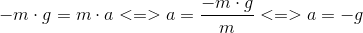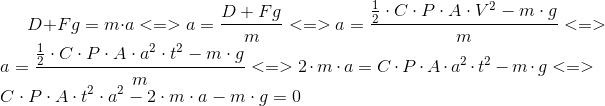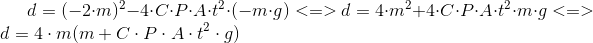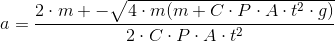User Name Remember Me? Password

 Physics Physics Forum

 August 11th, 2018, 03:45 AM #1 Senior Member   Joined: Oct 2015 From: Greece Posts: 116 Thanks: 8 Analyzing Drag Force And Free Falling. Are my calculations correct? I know that if two objects (starting at the same time) are free falling without a drag force being applied to them, the acceleration is:Which means it does not depend on the mass of the object. So the two objects no matter their mass, will accelerate with the same magnitude and they will reach the ground at the same time. On Earth,if you see two objects free falling, the heavier one will accelerate faster. So let's see what maths can tell us by applying Newton's second law: C = coefficient of air's drag force. P = air's density A = the area of the free falling body. V = the velocity of the free falling body. D = Drag force. Fg = body's weight. Be aware that we don't have an initial velocity.So if we assume that we are leaving two objects with the same Area (A) (a metal block and a leaf) and the metal's mass >> leafs mass , the above formula explains why the metal block will accelerate faster (numerator is big if m is big so does the acceleration). The only think i can't understand in this formula is why i have two solutions (well i forgot to check when d = 0 or d < 0 or d > 0). So if we say d > 0 we have the above formula with two solutions for acceleration. Can i reject one of them and why? I can't see that... Last edited by babaliaris; August 11th, 2018 at 03:48 AM.August 11th, 2018, 05:51 AM #2 Senior Member   Joined: Oct 2015 From: Greece Posts: 116 Thanks: 8 Guys forget it. Unfortunately because in this situation acceleration is not constant i can't use v=vo+at , so my analysis is wrong. I will come back and re-attempt to solve this when i reach the chapter about motion with varying acceleration.September 3rd, 2018, 08:06 AM   #3
Senior Member

Joined: Apr 2014
From: Glasgow

Posts: 2,155
Thanks: 731

Math Focus: Physics, mathematical modelling, numerical and computational solutions
Quote:
 Originally Posted by babaliarisGuys forget it. Unfortunately because in this situation acceleration is not constant i can't use v=vo+at , so my analysis is wrong. I will come back and re-attempt to solve this when i reach the chapter about motion with varying acceleration.
No problem. Air resistance is actually a non-trivial problem with very interesting maths and physics.

In a lot of preliminary texts, air resistance is assumed to be proportional to the velocity. However, the truth is that it is probably closer to velocity squared. However, because air resistance depends so much on the object's shape, the dependence is not straightforward.

In general, the main approach is to do the following:

1. Do a force balance on the object undergoing motion
2. Model the forces as a function of time
3. Develop an equation of motion based on the forces at a given point in time
4. Solve the equation of motion using boundary conditions

So, let's consider three cases for a simple example: an apple falling from a tree.

Case 1: no air resistance
Case 2: air resistance proportional to velocity
Case 3: air resistance proportional to velocity squared

Case 1: There is only one force, gravity, which is equal to $\displaystyle mg$ Therefore

$\displaystyle F = mg = ma$

$\displaystyle a = g$

$\displaystyle v = gt +c_1$

$\displaystyle x = \frac{gt^2}{2} + c_1t + c_2$

Introduce one boundary condition to get velocity and two to get displacement.

These are basically your SUVAT equations because the acceleration is constant.

Case 2: There are two forces, gravity ($\displaystyle mg$) and air resistance ($\displaystyle kv$)

$\displaystyle F = mg - kv = ma$

$\displaystyle a + \frac{k}{m}v = g$

$\displaystyle \frac{dv}{dt} + \frac{k}{m}v - g = 0$

This is a linear first-order ODE that can be solved analytically using the integrating factor method. This one is not too bad.

Case 3: There are two forces, gravity ($\displaystyle mg$) and air resistance ($\displaystyle kv^2$)

$\displaystyle F = mg - kv^2 = ma$

$\displaystyle a + \frac{k}{m}v^2 = g$

$\displaystyle \frac{dv}{dt} + \frac{k}{m}v^2 - g = 0$

This is a non-linear first-order ODE. Usually people use numerical integration for this one.

It only gets more complicated from there...

Have fun!Tags analyzing, drag, falling, force, freeSearch tags for this page

### plotting independent components of ICA in matlab

Click on a term to search for related topics.
 Thread ToolsShow Printable VersionEmail this Page Display ModesLinear ModeSwitch to Hybrid ModeSwitch to Threaded ModeSimilar Threads Thread Thread Starter Forum Replies Last Post shunya Elementary Math 1 December 13th, 2015 03:43 AM Kinroh Physics 3 January 9th, 2015 06:42 PM leo255 Calculus 1 December 16th, 2014 07:48 PM flower555 Calculus 1 October 16th, 2010 04:05 PM ask2 Calculus 2 November 13th, 2009 02:08 AM

 Contact - Home - Forums - Cryptocurrency Forum - Top

Copyright © 2019 My Math Forum. All rights reserved.